# From Optimization to Regret Minimization and Back Again

Ioannis Avramopoulos1, Jennifer Rexford2, and Robert Schapire2
Deutsche Telekom Laboratories1and Princeton University2

### Abstract:

Internet routing is mostly based on static information--it's dynamicity is limited to reacting to changes in topology. Adaptive performance-based routing decisions would not only improve the performance itself of the Internet but also its security and availability. However, previous approaches for making Internet routing adaptive based on optimizing network-wide objectives are not suited for an environment in which autonomous and possibly malicious entities interact.

In this paper, we propose a different framework for adaptive routing decisions based on regret-minimizing online learning algorithms. These algorithms, as applied to routing, are appealing because adopters can independently improve their own performance while being robust to adversarial behavior. However, in contrast to approaches based on optimization theory that provide guarantees from the outset about network-wide behavior, the network-wide behavior if online learning algorithms were to interact with each other is less understood. In this paper, we study this interaction in a realistic Internet environment, and find that the outcome is a stable state and that the optimality gap with respect to the network-wide optimum is small. Our findings suggest that online learning may be a suitable framework for adaptive routing decisions in the Internet.

# 1 Introduction

The global flow of Internet traffic depends on the interaction of independent networks that interconnect through routing protocols to deliver the traffic. The routing decisions are based for the most part on static information (such as number of hops to destination or business relationships with neighboring networks) ignoring dynamic performance metrics. Making routing decisions adaptive would not only improve the performance but also the security of the global routing system by enabling the end systems to route around adversaries .

Previous approaches to add adaptivity to routing decisions have been mostly based on optimization theory. These efforts date back to the work of Gallager  and have received significant attention since then (see, for example, [9,18,16]). However, such optimal routing algorithms rely on trust (assuming, for example, that routers provide feedback about performance in a truthful manner) and seek to optimize network-wide objectives possibly at the expense of the performance of individual flows. In the Internet, an adversary can exploit assumptions of trust to disrupt communication, and, therefore, Internet routing should be robust to such adversarial behavior. Furthermore, in the general interdomain setting, individual networks are not likely to seek to optimize a network-wide objective but rather their own performance.

In this paper, we propose a different framework for adding adaptivity to routing decisions, one based on the substantial body of work in learning theory and game theory on algorithms for making repeated decisions that aim to minimize regret. The regret of an algorithm is the difference between the performance of the sequence of decisions generated by the algorithm and the performance of the best fixed decision in hindsight. Several decision-making algorithms have been proposed that approach zero regret even against a fully adaptive adversary (e.g., [19,2]). The problem of routing data traffic between a source and a destination node over a set of paths can be cast as a problem of repeated decision making in which the routing algorithm must decide in a repeated fashion over which paths to forward the traffic.

Casting the problem of routing as a decision-making problem enables us to leverage recent theoretical results on regret minimization to develop a framework for making routing decisions adaptive. This framework is able to address the disadvantages of optimization-theory-based approaches. The reason is that a zero-regret routing algorithm as employed by a single source-destination pair is able to match the performance of the best path between source and destination irrespective of how the remaining pairs behave. This property is compatible with the incentives of rational adopters that prioritize optimizing their own performance over centrally designed network-wide objectives. Furthermore, the performance of the best path is matched even against a fully adaptive adversary that controls routers in a subset of the paths and behaves maliciously to disrupt communication. Using regret minimization, this disruption is prevented as long as an adversary-free path exists.

However, although previous work on regret minimization has developed algorithms that make these guarantees possible, it has largely neglected the question of what the network-wide behavior of the system would be if zero-regret routing algorithms were to interact with each other. Note that although it is certainly true that each source-destination pair is able to match the performance of the best path, it is nevertheless important to demonstrate that good performing paths do in fact exist. This is important for deployment in Internet environments where achieving the ability to counter adversaries should not affect the efficiency during normal operation. In this paper we seek to answer the question of what the network-wide behavior of a system of interacting zero-regret algorithms is through a realistic simulation study using data collected from the Internet2 backbone network.

Our findings can be summarized along two dimensions. First, we find that the outcome of the interaction is a stable state, which agrees with previous theoretical results derived in the Wardrop model of infinite traffic sources controlling infinitesimal amounts of traffic . This model differs, however, from ours in that we consider finite traffic demands. Second, we find that performance at the equilibrium is close to optimal. This result is in contrast to previous theoretical results on the price of total anarchy that predict a large optimality gap in the worst case . Furthermore, our findings assume that the routing algorithms only have access to end-to-end measurements and do not rely on feedback from intermediate nodes. Our results suggest that regret minimization is aptly positioned to drive Internet routing decisions.

# 2 Regret minimization

## 2.1 Online decision problems

An online decision problem can be formulated as a repeated game between a decision maker and the environment . The game proceeds in rounds, and at each round (or time step)of the time horizon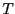, the decision maker must choose a probability distributionover a set of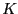actions. Then the environment (that is possibly controlled by an adversary) chooses one rewardfor each action. The actionof the decision maker is drawn according to distribution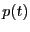and the decision maker receives reward.

The gain of actionis the sum of the action's rewards over the time horizon, i.e.,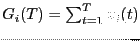, and the gain of the decision maker is the sum of the received rewards over the time horizon, i.e.,. The regret of the decision maker is defined as(often normalized by dividing by). The goal of the decision maker is to minimize the regret, and approach the gain of the best action.

In the course of the game, the decision maker gathers and uses as input information about the environment. Performance depends on the amount of information that can be gathered. In the full information setting, after a decision is made at each time stepthe decision maker observes. That is, access is given not only to the rewards that were received because of the actions that were taken but also to the rewards that would have been received if alternate actions had been taken. In the multi-armed bandit setting, at each time stepthe decision maker only observes the reward for the action that was actually taken. In both settings, there exist algorithms whose normalized regret approaches zero as the time horizon approaches infinity even if the rewards are generated by a fully adaptive adversary who controls the environment and is able to observe the decisions of the decision maker. In the full information setting, the regret is lower by roughly a factor proportional to.

## 2.2 Routing as an online decision problem

The problem of routing data traffic between a source node and a destination node in a network can be cast as a decision making problem as follows.

The decision maker is an independent instance of the routing algorithm (making routing decisions for the traffic between, say, a given pair of source and destination nodes), and the actions available correspond to the paths along which data packets can be forwarded. The probability distributionchosen at each time step determines the routing decisions for the data packets and essentially corresponds to how incoming traffic is split over theoutgoing paths. We call this probability vector the distribution vector. The routing algorithm must decide at each time step how to adjust the distribution vector. The time steps correspond to the instants that the algorithm can revise its decision. The reward for each decision corresponds to some performance metric such as packet loss, delay, or throughput. Such performance metrics can be estimated in practice through measurements (whether based on simple active probes or more secure measurement techniques [3,15]).

In modeling the decision process of the routing algorithm, we furthermore take into account the following in a realistic Internet environment. First, the decisions made by the routing algorithm have an impact on future rewards. This is true not only because of the time required to deliver packets from source to destination but also because of the interaction of the decisions made by different source-destination pairs. Because of this interaction, the response of the environment to the actions of the decision maker should not be considered independent of, or oblivious to, those actions (as has been assumed by a significant body of work on regret minimization) but rather dependent on them, i.e., adaptive.

Second, we make the following observations about the amount of information that is available to the routing algorithm. The full information setting assumes that the decision maker has access to the rewards that would have been obtained if alternate decisions had been made. However, standard measurement techniques cannot provide this information, i.e., they are not able to predict the performance that would have been observed if the data traffic had been forwarded over an alternate path. Therefore, in a realistic environment, the routing algorithm may only learn the rewards for the specific actions that were taken, which corresponds to the bandit setting. In the remainder of this paper, we only consider this latter setting and, furthermore, assume that performance estimates are obtained through end-to-end measurements. It is worth noting that because in practice the numberof paths available to the routing algorithm are limited and because the regret in the bandit setting is roughlytimes worse than the regret in the full information setting, performance under both settings is comparable.

## 2.3 Bandit-based routing algorithmThe learning algorithm we consider in this paper is algorithm Exp3  (Figure 1). The algorithm proceeds in rounds. At each rounda probability distributionis selected over the paths and a routing decisionis made for one unit of traffic demand (e.g., a packet). The outcome of the decision is a rewardthat is used to update labels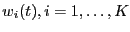according to formula (2). In the next round, these labels are used to recompute the distributionaccording to formula (1). Note that the probabilityof selecting pathdepends not only on past performance but also on a random component determined by parameter. This parameter controls a tradeoff between exploration and exploitation. If, the algorithm only explores (and does not exploit). If, the algorithm only exploits. If, the normalized regret of Exp3 provably approaches 0 asapproaches.

# 3 Interaction in Artificial Setting

To study the optimality and stability of zero-regret routing algorithms, our evaluation is based on an artificial and a realistic setting. The artificial setting provides a minimal environment in which to study the interaction of adaptive routing decisions, whereas the realistic setting is based on a more accurate model of an Internet environment. We start with the artificial setting in which we are able to show a positive result that motivates our investigation in the realistic setting.## 3.1 Methodology

Network topology and traffic demands: In the artificial topology, shown on the right of Figure 2, there are three source nodesthat must simultaneously send a unit of traffic each to destination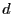. Each source is able to access the destination through three alternate paths each crossing one of the nodes. We assume that all links have unit capacity and, therefore, links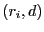are the bottleneck links.

Simulation setup: The simulation proceeds in steps (or rounds). At the beginning of a round each sourcedecides according to algorithm Exp3 along which path to forward its unit traffic demand (e.g. one packet). Then the load on each link is determined. Congestion is modeled as follows. If the load of a link is one, the load is successfully delivered to the next hop. However, if the load exceeds one, then one randomly selected unit of demand is successfully delivered but the rest of the demands are discarded. At the end of the round, the traffic sources are able to determine whether their demands were successfully delivered. Based on this feedback, the distribution vector of Exp3 is updated assuming a reward of one if the delivery is successful and a reward of zero otherwise.

Performance metrics: In this artificial setting, we measure performance by counting the number of traffic units that are successfully delivered to the destination. Given the small size of the network, to study the stability of the interaction, we simply plot the distribution vectors as a function of the simulation step.

## 3.2 EvaluationFigure 3 shows the probability of selecting each path in this artificial setting as a function of the simulation step. After a transient period of exploration, the routing processes converge to an outcome in which sources,, andselect paths,, and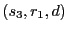respectively with very high probability (and the remaining paths with very low probability). This corresponds to a close-to-optimal network-wide outcome in which the probability of delivering three traffic units to the destination is very high. Furthermore, this outcome is stable in that the distribution vectors converge to a fixed distribution. This behavior was observed for a wide range of values of parameter. It is worth noting that the routing processes do not converge to fixed routing decisions--every combination of decisions has a non-zero probability of arising. This is typical for online learning algorithms that always explore alternate possibilities.

# 4 Interaction in Realistic Setting

## 4.1 Methodology

Network topology and traffic demands: To study adaptive routing in a realistic environment we use the Internet2 network (Figure 2) that provides Internet connectivity for research institutions in the US. In Internet2, the topology, routing, and traffic data are publicly available, enabling us to reproduce a realistic intradomain environment. Using these data we computed hourly traffic matrices during one week in May 2008. To a certain extent this environment also approximates an interdomain setting. An ideal approximation of an interdomain environment would correspond to each customer of Internet2 independently controlling its routing decisions. However, we found that data are not sufficient to calculate a traffic matrix at this granularity. Instead we compute router-to-router traffic matrices and assume that the traffic between each pair of routers is controlled by an independent process. In this way, we are able to study the interaction ofindependent flows. We leave a more thorough evaluation in general interdomain environments as future work.

We found that the network utilization from the traffic matrices calculated by our direct measurements was low. To also study adaptivity under conditions of high load we generated synthetic traffic matrices by scaling the real ones to achieve a maximum link utilization of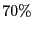.

Multiple paths between a source and destination were computed by successive shortest path computations in which at most one link was removed from the topology in an iterative fashion. The average number of paths for each source-destination pair is.

Simulation setup: The simulation proceeds in rounds. At the beginning of each round, each routing process splits its traffic demand according to its distribution vector and forwards it along the corresponding paths. That is, if the traffic demand for source-destination pairis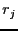and its distribution vector at roundis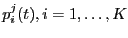, whereis the number of paths, then the amount of flow that the routing process forwards over pathis. (Note that this setup differs from the setup in the artificial setting in which the traffic demands were unsplittable. We have omitted our simulation results for unsplittable demands in the realistic setting in the interest of space as performance was generally inferior to the performance when the demands could be split. We have chosen though to retain the positive result of the artificial setting for its elegance.)

After computing the total flow on each link, the queuing delay is determined. If utilization does not exceed, the queuing delay is determined by the M/M/1 formula. However, similarly to , if utilization exceeds, the delay becomes proportional to the utilization according to a large constant. In this way, we can assign finite delay even to links whose utilization exceeds one.

Following the computation of the link delays, each routing process learns the end-to-end delay of each path to which it forwarded data traffic. Then each process updates its distribution vector by simulating algorithm Exp3 as if the demand consisted of discrete traffic units.

Performance metrics: The network-wide metric we use to evaluate performance is the average queuing delay over all links. We compare the average queuing delay incurred by interacting instances of the learning algorithm to the optimal average queuing delay (assuming multipath routing) that we computed using the cvx convex optimization software .

In the Internet2 network, evaluating stability by plotting the distribution vectors is unwieldy. Instead we evaluate stability by observing over time the differences'' between successive distribution vectors (and summing such differences over all source-destination pairs). The measure of difference we use is the Kullback-Leibler divergence, a standard information-theoretic measure of the difference between probability distributions. The KL-divergence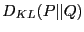between two probability vectorsandis given by the following formula:Note that as the difference betweenanddecreases so does, which becomes zero if.

Letbe the set of all source-destination pairs. For source-destination pair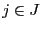letbe its distribution vector at round. Then our stability metric is the following sum.

## 4.2 EvaluationOptimality: In Figures 4 and 5, we compare the network queuing delay incurred by interacting instances of the learning algorithm with that of optimal routing by plotting the empirical cumulative distribution function (CDF) of the optimality gap. We define the optimality gap as the ratio of the average queuing delay of Exp3 over the optimal average queuing delay. In Figure 4, the ratios correspond to the traffic matrices from our measurements in the Internet2 network. In Figure 5, the traffic matrices from our measurements have been scaled so that the maximum link utilization under static shortest-path routing according to operator-selected IS-IS weights is. We plot the CDF of the optimality gap at the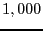th,th, and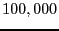th iteration. Observe that the optimality gap decreases with the iterations and almost vanishes atiterations. In fact, the optimality gap is a strictly decreasing function of the simulation step. This is shown in Figure 6 that plots the optimality gap on a semilogarithmic scale as a function of the simulation step for one particular traffic matrix (noting that the behavior in the figure is typical).

Translating simulation steps into real time depends on the measurement methodology. For example, if measurements are performed through randomly sampling inbound packets, each iteration corresponds to the time between successive samples implying that the time lapse between iterations is on the order of a few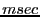. Therefore, assuming an iteration inverval of, the optimality gap shrinks in less than(iterations) and becomes negligible in less than(iterations) as shown in Figure 4.

In summary, the performance of Exp3 is close to optimal under normal operation while at the same time Exp3 is able to counter malicious attacks, a property that previous optimal routing algorithms do not have.

It is worth noting that although Exp3 is designed to match the performance of the best path between source and destination, in our simulation, its performance approaches that of the best distribution vector. We have verified that in our setting the gap between between optimal single-path and multipath routing is small. It is an interesting question whether Exp3 can compete against the best distribution vector in other Internet environments.Stability: Figure 6 plots the previously defined stability metric on a logarithmic scale as a function of the iteration step for one traffic matrix (noting that the behavior in the figure is typical). The figure shows that the stability metric is a strictly decreasing function of the simulation step (that, in fact, drops about four orders of magnitude in the duration of the simulation), implying that the distribution vectors converge to a fixed distribution. Observe that the changes in the distribution vector from one iteration to the next have a diminishing effect on the network cost that becomes negligible after the cost reaches a plateau.

# 5 Related work

Traffic engineering: Traffic engineering refers to the process by which routing adapts to the traffic demands and can be performed either offline (e.g., see  for a survey) or online (e.g., [18,11]). Related to this paper are online traffic engineering protocols. However, these protocols are not resilient to attacks by compromised routers. Furthermore, as they are designed for deployment inside a routing domain, they are not general enough to be applicable to interdomain settings.

Regret minimization: From the perspective of an individual player, regret minimization has been studied in the general repeated game setting (e.g., [19,2,8]), and in more specific routing games. In such games, an algorithm must repeatedly choose a path between source and destination assuming an adversary controls the edge costs. Zero-regret algorithms in this setting appear in [17,5]. These studies laid the foundation for the approach proposed in this paper, but are not concerned with the stability or optimality of the interaction of zero-regret algorithms. A routing algorithm that minimizes regret from the perspective of a single source-destination pair is presented in , but this algorithm is not studied when multiple source-destination pairs interact.

Interaction of regret-minimizing algorithms: Previous work has studied whether zero-regret algorithms in a repeated game setting converge to a Nash equilibrium. In two-player zero-sum games, the outcome of the interaction is a minimax solution . However, in general games, a Nash equilibrium may not be approachable in polynomial time by any polynomial algorithm . Positive stability results are known for routing games in the Wardrop model of an infinite number of traffic sources each controlling infinitesimal traffic, in which convergence to a Nash equilibrium is shown in . Different from this work, we consider finite traffic demands.

The optimality of the interaction of zero-regret algorithms is studied in  where it is shown that in the worst-case there is a high price in moving from centralized to independent routing decisions. Our findings suggest that, in practice, the optimality gap is small. Small optimality gap under selfish routing was previously observed in a related study on the price of anarchy in realistic Internet environments . This study measured the optimality gap of Nash equilibria whereas we study the interaction of regret minimization algorithms.

# 6 Conclusion

In this paper, we proposed online learning algorithms as a framework for adding adaptivity to routing decisions and studied how such algorithms interact in a realistic Internet environment. We found that the outcome of the interaction is a stable state and that the optimality gap with respect to the network-wide optimum is small. We conclude that online learning may be a suitable framework for routing in the Internet.

# Acknowledgments

This project has benefited from data collected in the Internet2 Observatory Project. We would like to thank Elliott Karpilovsky and the anonymous reviewers for their insightful comments. Ioannis Avramopoulos was with Princeton University while performing part of this work.

## Bibliography

1
https://www.stanford.edu/~boyd/cvx/.

2
P. Auer, N. Cesa-Bianchi, Y. Freund, and R. E. Schapire.
The non-stochastic multi-armed bandit problem.
SIAM Journal on Computing, 32(1):48-77, 2002.

3
I. Avramopoulos and J. Rexford.
Stealth probing: Efficient data-plane security for IP routing.
In Proc. USENIX Annual Technical Conference, May/Jun. 2006.

4
B. Awerbuch, D. Holmer, H. Rubens, and R. Kleinberg.
In Proc. IEEE Infocom, Mar. 2007.

5
B. Awerbuch and R. Kleinberg.
Online linear optimization and adaptive routing.
Journal of Computer and System Sciences, 74(1):97-114, 2008.

6
A. Blum, E. Even-Dar, and K. Ligett.
Routing without regret: On convergence to Nash equilibria of regret-minimizing algorithms in routing games.
In Proc. PODC, Jul. 2006.

7
A. Blum, M. Hajiaghayi, K. Ligett, and A. Roth.
Regret minimization and the price of total anarchy.
In Proc. STOC, May 2008.

8
N. Cesa-Bianchi and G. Lugosi.
Prediction, Learning, and Games.
Cambridge University Press, 2006.

9
M. Chiang, S. H. Low, A. R. Calderbank, and J. C. Doyle.
Layering as optimization decomposition: A mathematical theory of network architectures.
Proceedings of the IEEE, 95(1):255-312, 2007.

10
The complexity of computing a Nash equilibrium.
In Proc. STOC, May 2006.

11
S. Fischer, N. Kammenhuber, and A. Feldmann.
REPLEX--Dynamic traffic engineering based on Wardrop routing policies.
In Proc. CoNEXT, Dec. 2006.

12
B. Fortz, J. Rexford, and M. Thorup.
Traffic engineering with traditional IP routing protocols.
IEEE Communication Magazine, 40(10):118-124, 2002.

13
Y. Freund and R. E. Schapire.
Adaptive game playing using multiplicative weights.
Games and Economic Behavior, 29:79-103, 1999.

14
R. Gallager.
A minimum delay routing algorithm using distributed computation.
IEEE Transactions on Communications, 25(1):73-85, 1977.

15
S. Goldberg, D. Xiao, E. Tromer, B. Barak, and J. Rexford.
Path-quality monitoring in the presence of adversaries.
In Proc. ACM SIGMETRICS, Jun. 2008.

16
J. He, M. Suchara, M. Bresler, J. Rexford, and M. Chiang.
Rethinking internet traffic management: From multiple decompositions to a practical protocol.
In Proc. CoNEXT, Dec. 2007.

17
A. Kalai and S. Vempala.
Efficient algorithms for on-line optimization.
In Proc. COLT, Aug. 2003.

18
S. Kandula, D. Katabi, B. Davie, and A. Charny.
Walking the tightrope: Responsive yet stable traffic engineering.
In Proc. ACM SIGCOMM, Aug. 2005.

19
N. Littlestone and M. K. Warmuth.
The weighted majority algorithm.
Information and Computation, 108(2):212-261, 1994.

20
L. Qiu, Y. R. Yang, Y. Zhang, and S. Shenker.
On selfish routing in Internet-like environments.
IEEE/ACM Transactions on Networking, 14(4):725-738, 2006.

21
D. Wendlandt, I. Avramopoulos, D. Andersen, and J. Rexford.
Don't secure routing protocols, secure data delivery.
In Proc. ACM SIGCOMM HotNets Workshop, Nov. 2006.

From Optimization to Regret Minimization and Back Again

This document was generated using the LaTeX2HTML translator Version 2002-2-1 (1.71)

Copyright © 1993, 1994, 1995, 1996, Nikos Drakos, Computer Based Learning Unit, University of Leeds.
Copyright © 1997, 1998, 1999, Ross Moore, Mathematics Department, Macquarie University, Sydney.

The command line arguments were:
latex2html -split 0 -show_section_numbers -local_icons -no_navigation regret.tex

The translation was initiated by Ioannis Avramopoulos on 2008-11-21

Ioannis Avramopoulos 2008-11-21